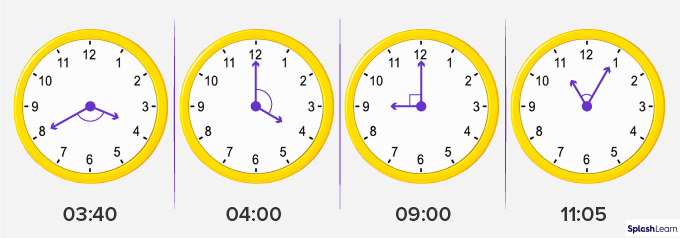# Perpendicular Lines – Definition with Examples

## Definition of Perpendicular Lines

In geometry, perpendicular lines are defined as two lines that meet or intersect each other at right angles (90°). The term ‘perpendicular’ originated from the Latin word ‘perpendicularis,’ meaning a plumb line.

If two lines, AB and CD, are perpendicular, then we can write them as AB ⟂ CD. The symbol ⟂ is used to indicate that the lines are perpendicular.

## Examples of Perpendicular Lines in Real Life

We can observe many perpendicular lines in real life. Some examples are shown below.

## Properties of Perpendicular Lines

The two main properties of perpendicular lines are given below.

1. These lines always intersect at right angles.
2. If two lines are perpendicular to the same line, they are parallel to each other and will never intersect.

## Constructing Perpendicular Lines

We can draw perpendicular lines in the following ways.

Using a Protractor

• Draw a horizontal line, PQ, on a sheet of paper.
• Mark a point, A, on it. This would be the point of intersection.
• Place the center of the protractor on Point A. Align the baseline of the protractor with the line PQ.
• At the 90° mark on the protractor, mark Point B. It is at the very top of the protractor.
• Join points A and B to get AB ⟂ PQ.

Using a Compass

• Draw a line, PQ, using a ruler. Mark a point A on it.
• Draw an arc of any radius on either side of Point A. The arc length should be the same on both sides.
• Mark the points where the arcs intersect the line as X and Y.
• Place the compass needle at point X and set a radius greater than XA. Draw an arc from X in the upper part of the line PQ.
• Keep the radius the same and cut an arc from Point Y, intersecting the previous arc at B.
• Join A and B using a ruler. Extend it if needed. The lines AB and PQ are perpendicular to each other.

Conclusion
Give your children opportunities to observe perpendicular lines in objects or places around them, such as a tall tree on the ground, an electric pole on the pavement, railway intersection, the corner of two adjacent walls, and high buildings.

If you wish to explore the meaning, history, or tips to excel in any topic, SplashLearn presents an entertaining platform for you. Read the informational blog posts to gain knowledge and practice with fun and entertaining educational games. Start learning today!

## Solved Examples

Example 1 If AB ⊥ CD and CD ⊥ EF. What can you say about AB and EF?

Line AB is perpendicular to line CD.

Also, line CD is perpendicular to line EF.

When two lines are perpendicular to the same line, then the two lines are parallel to each other.

Thus, lines AB and EF are parallel to each other.

Example 2: Are these lines perpendicular?

No, these lines are not perpendicular because they do not intersect at right angles.

Example 3: Are these lines perpendicular?

Yes, these are perpendicular lines. They intersect at 90°.

## Practice Problems

1

### At what time will the hands of the clock be perpendicular?

03:40 pm
04:00 pm
09:00 am
10:10 am
CorrectIncorrect
The hands of the clock intersect at right angles when the time is 9:00 am.2

### Perpendicular lines intersect each other at _____ angle.

right
obtuse
acute
straight
CorrectIncorrect
Perpendicular lines intersect each other at 90°. 90° angles are known as right angles.
3

Ball
Birthday Hat
Banana
Laptop
CorrectIncorrect